Publicité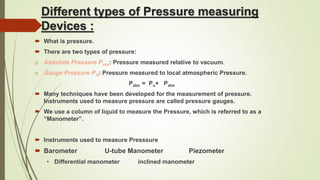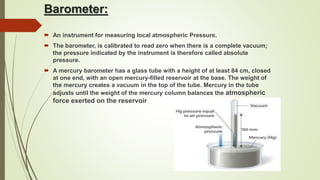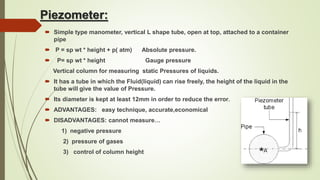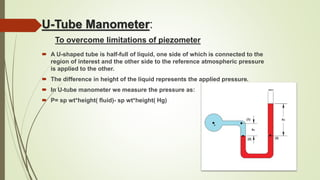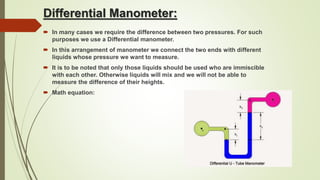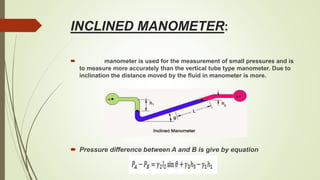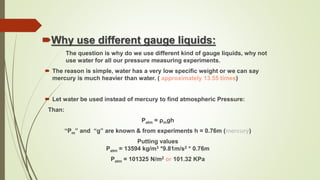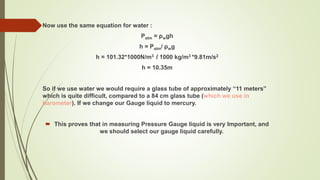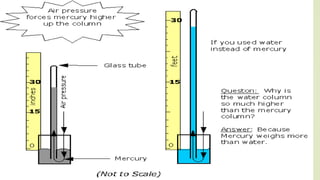1 sur 10
Publicité

### Fluid mechanics

1. Different types of Pressure measuring Devices :  What is pressure.  There are two types of pressure: o Absolute Pressure Pabs: Pressure measured relative to vacuum. o Gauge Pressure PO: Pressure measured to local atmospheric Pressure. Pabs = Po+ Patm  Many techniques have been developed for the measurement of pressure. Instruments used to measure pressure are called pressure gauges.  We use a column of liquid to measure the Pressure, which is referred to as a “Manometer”.  Instruments used to measure Presssure  Barometer U-tube Manometer Piezometer • Differential manometer inclined manometer
2. Barometer:  An instrument for measuring local atmospheric Pressure.  The barometer, is calibrated to read zero when there is a complete vacuum; the pressure indicated by the instrument is therefore called absolute pressure.  A mercury barometer has a glass tube with a height of at least 84 cm, closed at one end, with an open mercury-filled reservoir at the base. The weight of the mercury creates a vacuum in the top of the tube. Mercury in the tube adjusts until the weight of the mercury column balances the atmospheric force exerted on the reservoir
3. Piezometer:  Simple type manometer, vertical L shape tube, open at top, attached to a container pipe  P = sp wt * height + p( atm) Absolute pressure.  P= sp wt * height Gauge pressure Vertical column for measuring static Pressures of liquids.  It has a tube in which the Fluid(liquid) can rise freely, the height of the liquid in the tube will give the value of Pressure.  Its diameter is kept at least 12mm in order to reduce the error.  ADVANTAGES: easy technique, accurate,economical  DISADVANTAGES: cannot measure… 1) negative pressure 2) pressure of gases 3) control of column height
4. U-Tube Manometer: To overcome limitations of piezometer  A U-shaped tube is half-full of liquid, one side of which is connected to the region of interest and the other side to the reference atmospheric pressure is applied to the other.  The difference in height of the liquid represents the applied pressure.  In U-tube manometer we measure the pressure as:  P= sp wt*height( fluid)- sp wt*height( Hg)
5. Differential Manometer:  In many cases we require the difference between two pressures. For such purposes we use a Differential manometer.  In this arrangement of manometer we connect the two ends with different liquids whose pressure we want to measure.  It is to be noted that only those liquids should be used who are immiscible with each other. Otherwise liquids will mix and we will not be able to measure the difference of their heights.  Math equation:
6. INCLINED MANOMETER:  manometer is used for the measurement of small pressures and is to measure more accurately than the vertical tube type manometer. Due to inclination the distance moved by the fluid in manometer is more.  Pressure difference between A and B is give by equation
7. Why use different gauge liquids: The question is why do we use different kind of gauge liquids, why not use water for all our pressure measuring experiments.  The reason is simple, water has a very low specific weight or we can say mercury is much heavier than water. ( approximately 13.55 times)  Let water be used instead of mercury to find atmospheric Pressure: Than: Patm = ρmgh “Ρm” and “g” are known & from experiments h = 0.76m (mercury) Putting values Patm = 13594 kg/m3 *9.81m/s2 * 0.76m Patm = 101325 N/m2 or 101.32 KPa
8. Now use the same equation for water : Patm = ρwgh h = Patm/ ρwg h = 101.32*1000N/m3 / 1000 kg/m3 *9.81m/s2 h = 10.35m So if we use water we would require a glass tube of approximately “11 meters” which is quite difficult, compared to a 84 cm glass tube (which we use in barometer). If we change our Gauge liquid to mercury.  This proves that in measuring Pressure Gauge liquid is very Important, and we should select our gauge liquid carefully.
9. Thank you.
Publicité